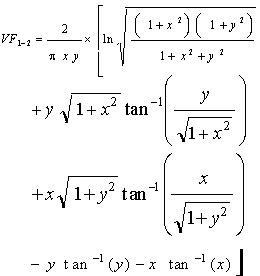Close

This page calculates the net radiant heat exchange for two isothermal (constant temperature) parallel flat black plates of equal size.The heat flow (q) from the plate is calculated as:

q = 56.69x10-9 x VF(1-2) x A x (T14 - T24)

Where 56.69x10-9 is the average Stefan-Bolzmann constant, A is the area of the plate, T1 is the plate 1 temperature and T2 is the plate 2 temperature. The view factor VF(1-2) (also known as radiation shape factor, angle factor, and configuration factor) is defined as:Where x and y are defined as:

x = L / D
y = W / D

Where L is the plate length, W is the plate width, and D is the distance between the plates.

References

Ellison G. N., Thermal Computations for Electronic Equipment, Van Nostrand Reinhold Company, New York, 1984. p. 78 - 79.

Holman, J.P., Heat Transfer, 7th ed., McGraw Hill Book Company, New York, 1990. p. 385 - 405.